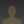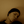0%
0%

Given almost no budget and some salvaged parts, the aim is to try and build a robot hopefully more advanced than simple avoidance.

Similar projects worth following
2.6k views
Ignoring the cost of the arduino UNO, I'm planning on keeping the costs below £10 (about \$17).
So far i've salvaged a couple of drive motors with gearboxes and wheels already attached.

Luckily China is an excellent source for very cheap (although not amazing quality) sensors, and parts. And so far i've got a basic sonar system set up using nothing more than an ultrasonic sensor, and servo costing little more than £1.

More photos to follow, next step mounting the sonar to the wheel base.

```

//Rangefinder Arduino code

//Ultrasonic Rangefinder
#include <PWMServo.h>
#include <NewPing.h>

PWMServo servo1;

unsigned int duration;

long timer;

#define svomin 10
#define svomax 150
#define svodormant 80

#define lmotor 1
#define rmotor 2

#define mStop 0
#define mForward 1
#define mReverse 2
#define mLeft 3
#define mRight 4

#define lmotorf 3
#define lmotorr 2
#define rmotorf 5
#define rmotorr 4

#define trigPin 14
#define echoPin 15
#define button1 16
#define avoid 17

#define maxdistance 100

long runTime = 0;
int robotState = mStop;
int mbm = 52;
int svo = svomin;
int count = svomax - svomin;
float distance;
int buttonState;
int lastButtonState = LOW;
int scanState = false;
int avoidState;
int lastAvoidState = LOW;
long lastDebounceTime = 0;
long debounceDelay = 50;

NewPing sonar(trigPin, echoPin, maxdistance);

void setup() {
servo1.attach(SERVO_PIN_A);
pinMode(trigPin, OUTPUT);
pinMode(echoPin, INPUT);
pinMode(button1, INPUT);
pinMode(avoid, INPUT);
pinMode(lmotorf, OUTPUT);
pinMode(lmotorr, OUTPUT);
pinMode(rmotorf, OUTPUT);
pinMode(rmotorr, OUTPUT);
Serial.begin(57600);
//Serial.print("AT+BAUD7");
svo = svodormant;
servo1.write(svo);
delay(1000);
timer = millis() + mbm;
scanState = false;
}

void loop() {

if (scanState == true) {
robotStop();
}

if (avoidState == LOW && scanState == false && robotState == mForward) {
scanState = true;
svo = svomin;
servo1.write(svo);
}

lastDebounceTime = millis();
}

if((millis() - lastDebounceTime) > debounceDelay) {
if(buttonState == HIGH) {
scanState = true;
svo = svomin;
servo1.write(svo);
}
}
}

if(timer < millis() && scanState==true) {
getDist2();
svo++;
if(svo > svomax) {
svo=svodormant;
smooth();
smooth();
Serial.println("");
Serial.println("BEGIN");
for(int i=0;i<=count;i++) {
Serial.print("S");
Serial.println(i+10,DEC);
Serial.print("V");
Serial.println(distance[i], DEC);
}
scanState = false;
robotThink();
}
servo1.write(svo);
if(svo==svomin) { delay(1000); }
timer = millis() + mbm;
}
if (runTime < millis()) {
motor(lmotor, mStop);
motor(rmotor, mStop);
robotThink();
}
}

void getDist2() {
duration = sonar.ping();
distance[svo-svomin] = duration/US_ROUNDTRIP_CM;
if(duration==0) { distance[svo-svomin] = maxdistance; }
}

void smooth() {

int p;
float temp;
float mean;

for(int i=0;i<=count;i++) {
mean=0;
for(int t=-4;t<=4;t++) {
p=i+t;
if(p>count) {
p=p-(count+1);
}
if(p<0) {
p=p+(count+1);
}
mean = mean + distance[p];
}
mean = mean / 9;
distance[i] = mean;
}
}

void motor(int m, int d) {
int mf;
int mr;
switch (m) {
case lmotor:
mf = lmotorf;
mr = lmotorr;
break;
case rmotor:
mf = rmotorf;
mr = rmotorr;
break;
default:
mf = 0;
mr = 0;
}
switch (d) {
case mStop:
digitalWrite(mf, LOW);
digitalWrite(mr, LOW);
break;
case mForward:
digitalWrite(mf, HIGH);
digitalWrite(mr, LOW);
break;
case mReverse:
digitalWrite(mf, LOW);
digitalWrite(mr, HIGH);
break;
default:
digitalWrite(mf, LOW);
digitalWrite(mr, LOW);
}
}

void robotStop() {
motor(lmotor, mStop);
motor(rmotor, mStop);
runTime = 1000;
robotState = mStop;
}

void robotForward() {
motor(lmotor, mForward);
motor(rmotor, mForward);
runTime = 1000;
robotState = mForward;
}

void robotReverse() {
motor(lmotor, mReverse);
motor(rmotor, mReverse);
runTime = 1000;
robotState = mReverse;
}

void robotLeft() {
motor(lmotor, mReverse);
motor(rmotor, mForward);
runTime = 1000;
robotState = mLeft;
}

void robotRight() {
motor(lmotor, mForward);
motor(rmotor, mReverse);
runTime = 1000;
robotState = mRight;
}

void robotThink() {
if (avoidState == HIGH && scanState == false) {
robotForward();
}
if (avoidState == LOW && scanState == false) {
robotRight();
}
if (avoidState == LOW && scanState...```

• 1 × Arduino Uno
• 1 × HC-SR04 based Ultrasonic Distance Sensor
• 1 × L9110S H Bridge Stepper Motor Dual DC Driver Controller Board Module for Arduino
• 1 × IR Obstacle Avoidance Sensor Module
• 2 × Salvaged motors and gearboxes
• ### FreeBasic Reciever Code

```'Freebasic Rangefinder Reciever Code

#Include "FBGFX.bi"
Using fb

ScreenRes 800,600,32

Const TRUE = 1
Const FALSE = 0

Const maxdistance = 100          'Maximum distance we want to read
Const factor = 250 / maxdistance     'scale factor for drawing bars
Const minang = 10            'Starting angle, as a cheap servo was used moving to 0 causes problems
Const maxang = 150            'Set to keep view in front of robot
Const totalang = maxang-minang
Const angfactor = 1

Const pi = 3.14159265359

Dim As UByte rec_Data
Dim As UByte bounce
Dim As UByte datastart

Dim As uByte fin, gotang, gotmessage

Dim As ubyte serialData
Dim As String message, marker

Dim As Integer ang, i
Dim As Double distance

Declare Function radang(ang As Double) As Double
Declare Sub draw_line(ang As Integer, distance As Double)

fin = FALSE
gotang = FALSE
gotmessage = FALSE
datastart = TRUE

Open com "COM14:57600,N,8,1,BIN,CS0,DS0" As 1        'Opens communication with serial port (in this case bluetooth)
Sleep 100

ang=0

Cls
Circle (400,300),255,RGB(196,0,0),,,,F
Line (0,301)-(800,600),RGB(0,0,0),bf
Do

If MultiKey(SC_ESCAPE) Then fin = TRUE

If LOC(1) > 0 Then
If datastart = TRUE Then
Cls
Circle (400,300),255,RGB(196,0,0),,,,F
Line (0,301)-(800,600),RGB(0,0,0),bf
datastart = FALSE
End If
Get #1, ,serialData
EndIf

If serialdata <> 10 Then message = message & Chr(serialdata)     'start to decode the information coming in
If serialdata = 10 Then
'Print message
If Left(message,5) = "BEGIN" Then                 'Start of data stream
datastart = TRUE
EndIf
If Left(message,1) = "S" Then                     'Angle Data
ang = Val(Right(message,Len(message)-1)) - 10
gotang = TRUE
EndIf
If Left(message,1) = "V" Then                     'Distance Data
distance = Val(Right(message,Len(message)-1))
gotmessage = TRUE
EndIf
message = ""
EndIf

If gotang = TRUE And gotmessage = TRUE Then                 'draw line on display if both angle and data recieved
gotang = FALSE
gotmessage = FALSE
If ang = 0 Then
EndIf
draw_line(ang,distance)
EndIf

For i = 0 To 250 Step 50
marker = Str(Int(i/factor))
Draw String (400-(4*Len(marker)),290-i), marker, RGB(255,255,255)
Next i
Line (399-totalang,350)-(401+totalang,400),RGB(0,0,255),b

Loop Until fin = TRUE
Close #1

End 0

Sub draw_line(ang As Integer, distance As Double)

Dim As Double x1,y1,x2,y2, fdist, anginc, i, inc, ang2
Dim As Integer x3, c, d

If distance = 0 Then distance = maxdistance
Static lastdist As Double

ang2 = 360 - ang - ((180-(totalang))/2)

fdist = distance * factor
inc = (lastdist - distance) /500
x1 = 400
y1 = 300
x3 = ((totalang-ang)*2)+ (400-(totalang))
c = (255-((distance/maxdistance) * 255))
If c < 0 Then c = 0
If c > 255 Then c = 255

For i = -1 To 0 Step 0.002
d = c + (inc*i)
If d > 255 Then d = 255

x2 = ((fdist + (inc*i)) * Cos(radang(ang2 + i))) + x1
y2 = ((fdist + (inc*i)) * Sin(radang(ang2 + i))) + y1

Line (x1,y1)-(x2,y2),RGB(0,0,0)
Line (x3 + i,350) - (x3 + i,400),RGB(d,d,d)
Next i

lastdist = distance
End Sub

Function radang(ang As Double) As Double

End Function
```

• ### Many Updates, One Log

Ok, so the first couple of images i posted showed no more than a sensor on a servo.  Since then i've put together a wheelbase (not the most efficient setup, but it serves a purpose for the moment), added a bluetooth connection, and an IR avoidance sensor.

The wheelbase is made from a couple of motors and gearboxes salvaged from an old robot toy.  Sadly these are massively power hungry, and so the drive batteries don't last very long at all.

I've used an uber cheap HC-06 bluetooth module from ebay for wireless communication, and although it was fussy at the start, it seems to stream the data nicely.  Sadly however i've not figured out a way of putting it to sleep when it's not in use, causing more of a power drain on the system.  Coupled with the cheap servo the arduino is also drawing it's fair share of current (although from a different set of batteries from the motors).

Next step is definitely to sort out the power issues.  Hopefully starting with the control side, and shutting down both the servo, bluetooth, and possibly even the ultrasonic sensor when they are not needed.

Share

## Discussions

zafer wrote 05/02/2015 at 20:58 point

Hello dude, are you still working on this project?  'Cause i've problem with my project. I'am working on arduino based sonar with stepper motor and hc-sr04 ultrasonic sensor, i wrote the codes on arduino and i am trying to processing radar screen on MatLab but it doesn't work. After 5-10 degree scanning, the scanner bar is missing every time. Can you send me your arduino codes for this project?

Are you sure? yes | no

sad_ken wrote 05/03/2015 at 07:41 point

I've not done anything to this for a while, my drive wheels were pretty poor so progress stalled.  Im happy to send you the code i have though.  My recieving code was written in freebasic, it is probably quite messy but hopefully you'll get the idea.

I'll try and dig out the code later today when i'm on a laptop, and post it here.

Are you sure? yes | no

davedarko wrote 08/05/2014 at 21:59 point
+skull for the Johnny 5 theme :)

Are you sure? yes | no

sad_ken wrote 08/06/2014 at 08:39 point
Thanks, I couldn't resist the addition after someone suggested it on the original photo.

Are you sure? yes | no

esot.eric wrote 12/10/2015 at 03:20 point

Hah, just came across this for the first time and as soon as I saw that image I thought "I bet davedarko would dig this"... well, I thought that *after* digging it, myself ;)

Are you sure? yes | no

## Similar Projects

Project Owner Contributor

### AVR Halloween Pumpkin V2Schupp

Project Owner Contributor

### SNES controller with FTDI.tehaxor69

Project Owner Contributor

### OSCAR: Omni Service Cooperative Assistant RobotPoh Hou Shun

# Does this project spark your interest?

Become a member to follow this project and never miss any updates